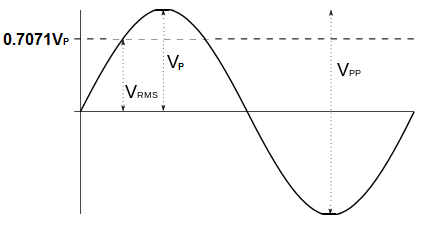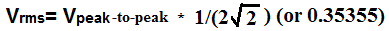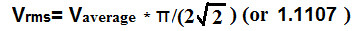﻿ RMS Voltage and Current- Explained ﻿# RMS Voltage and Current- ExplainedRMS, or root mean square (also called effective), voltage is a method of denoting a voltage sine waveform (AC waveform) as an equivalent voltage which represents the DC voltage value that will produce the same heating effect, or power dissipation, in circuit, as this AC voltage.

In other words, the waveform is an AC waveform, but the RMS value allows this waveform to be specified as if DC, because it is the equivalent DC voltage that delivers the same amount of power to a load in a circuit as the AC signal does over its cycle.

AC and DC waveforms can both represent voltage or current waveforms, but they are in different forms. AC waveforms fluctuate between positive and negative voltage in cycles. DC voltage is just constant one-way voltage that doesn't have cycles. Because of this difference, it's very difficult to compare the two.

This is where the RMS value is important. It gives us a standard to compare the amount of power that an AC waveform and a DC waveform can give to a circuit. RMS voltage is the DC equivalent of an AC waveform so that we can compare power dissipation with both the AC power and DC power waveforms. If we have a RMS waveform of an AC signal and it it is the same value as a DC waveform, then we know that both waveforms give off, or dissipates, the same amount of power in a circuit.

The reason RMS voltage is also called effective voltage is because it is just as effective as DC voltage in providing power to an element (it's equally effective). Since RMS voltage is the DC equivalent voltage, the RMS voltage is just as effective as its equivalent DC voltage in providing power to an element or load in a circuit.

### Examples of RMS Voltage

An example of RMS voltage is, let's say, we have a circuit with a load that we want to dissipate no more than 0.5 watts of energy from. This is the maximum constant power that this load can handle. If we're using a 10-volt DC power source, then the maximum current that can go through this circuit would be 50mA, because power= current x volts. Therefore, 10V x 50mA=0.5W. Now let's say, we want to switch with working from a DC circuit to working with an AC circuit. We can use this DC example that we have worked out so that we can also find the AC voltage that we can use for this circuit without surpassing its power limits. If, for this AC voltage, we also allow 50mA of current, then we know that we can use the RMS voltage of 10VAC to operate in this circuit. If we use an RMS voltage of 10V, then the peak voltage that we can use in this circuit is approximately 14.1V. This is how RMS voltage works. It allows us to work with DC circuit models, and we can find the appropriate AC voltage that causes the same heating or power dissipation through a load, as the DC voltage would.

Many types of voltages in real life are specified by their RMS values.

One prime example is the voltage from an AC outlet. You're probably familiar and know that 120V comes out from AC outlet, such as one that you would find at your home or any building in the United States. But you probably don't know what type of voltage this is? And the answer is, it is RMS voltage. The 120V is the RMS voltage. And the peak voltage for this is actually 170V. So the peak of this voltage is actually a whole lot bigger than 120V. And if you look at it from peak-to-peak, then the voltage from an AC outlet is actually 340V peak-to-peak.

By using the RMS voltage, the voltage that arises from an AC outlet, it more easily relates to DC circuits.

### How to Calculate RMS Voltage

The root-mean-square (RMS) voltage can be calculated either from the peak voltage, the peak-to-peak voltage, or the average voltage.

The formulas to calculate the RMS voltage from either of these voltages are shown below.

### How to Calculate RMS Voltage from Peak VoltageIf you are given the peak voltage value, you can calculate the RMS voltage using the above formula.

All you have to do to obtain the RMS value is to multiply the peak voltage by 0.7071.

### How to Calculate the RMS Voltage from Peak-to-Peak VoltageIf you are given the peak-to-peak voltage value, you can calculate the RMS voltage using the above formula.

All you must do to obtain the RMS value is to multiply the peak-to-peak voltage by 0.35355.

### How to Calculate the RMS Voltage from Average VoltageIf you are given the average voltage value, you can calculate the RMS voltage using the above formula.

All you must do to obtain the RMS value is to multiply the average voltage by 1.1107.

To use our online calculator to compute RMS voltage, see RMS Voltage Calculator.

Valuable Note- When using an oscilloscope to measure an AC signal, you can measure the peak value or peak-to-peak value of the AC waveform. To find the equivalent RMS value of this waveform, you would then only have to multiply that peak value by 0.707 or the peak-to-peak value by 0.35355.

Related Resources